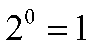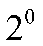If the index of any power function is zero, then the value of that function is

# If the index of any power function is zero, then the value of that function is

1. A
0
2. B
1
3. C
-1
4. D
None of these

Fill Out the Form for Expert Academic Guidance!l

+91

Live ClassesBooksTest SeriesSelf Learning

Verify OTP Code (required)

### Solution:

Concep- The exponent also referred to as the index or power, shows how many times the multiplication has been performed.
If the index of any power function is zero, the function's value will be 1.
For example,Here, the power function iswith an index zero.
Hence, the correct answer is option 2.

## Related content

 Area of Square Area of Isosceles Triangle Pythagoras Theorem Triangle Formula Perimeter of Triangle Formula Area Formulae Volume of Cone Formula Matrices and Determinants_mathematics Critical Points Solved Examples Type of relations_mathematics+91

Live ClassesBooksTest SeriesSelf Learning

Verify OTP Code (required)